# NCERT Solutions for Class 8 Maths Chapter 14 Exercise 14.4 – Factorisation

NCERT Solutions for Class 8 Maths Chapter 14 Exercise 14.4 – Factorisation, has been designed by the NCERT to test the knowledge of the student on the topic – Finding the Error

### NCERT Solutions for Class 8 Maths Chapter 14 Exercise 14.4 – Factorisation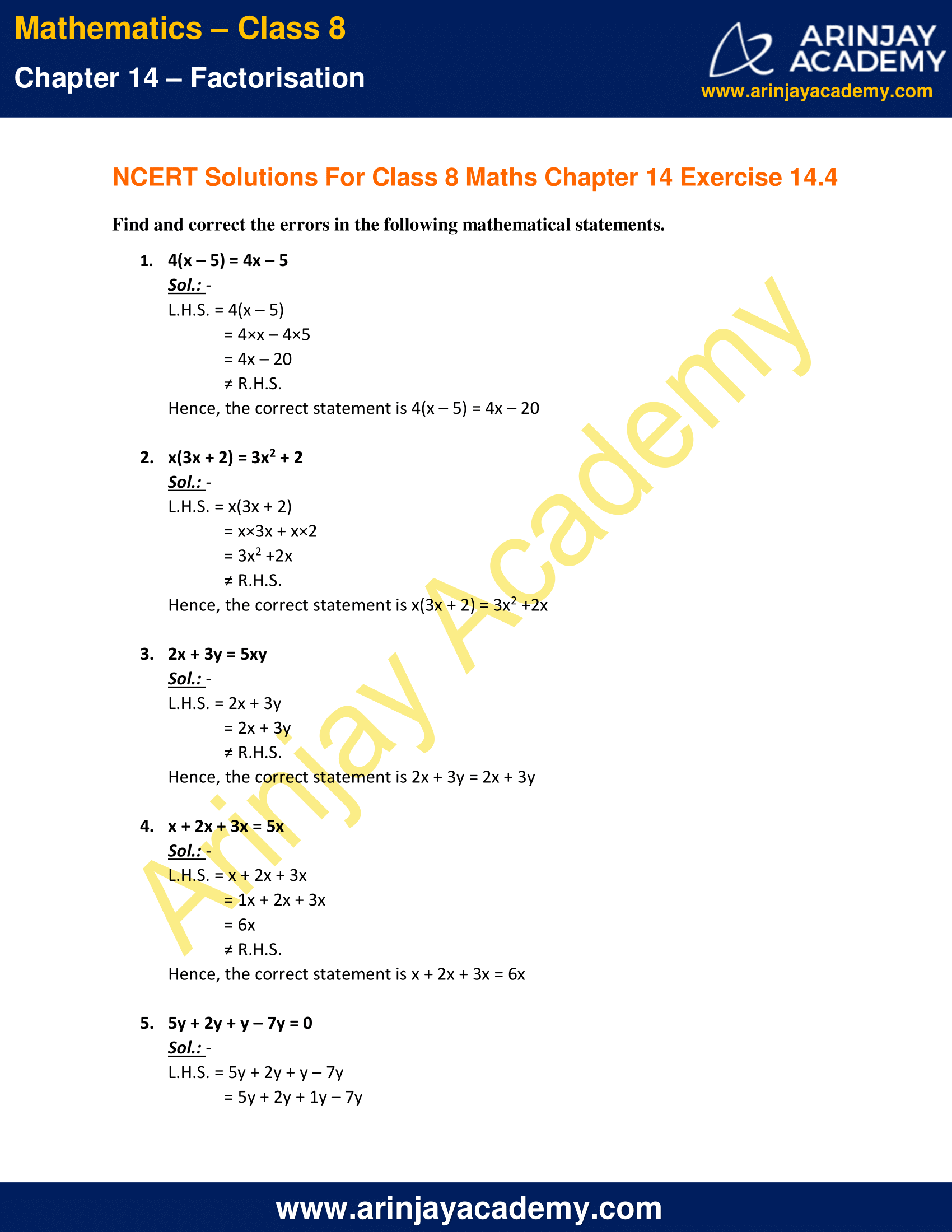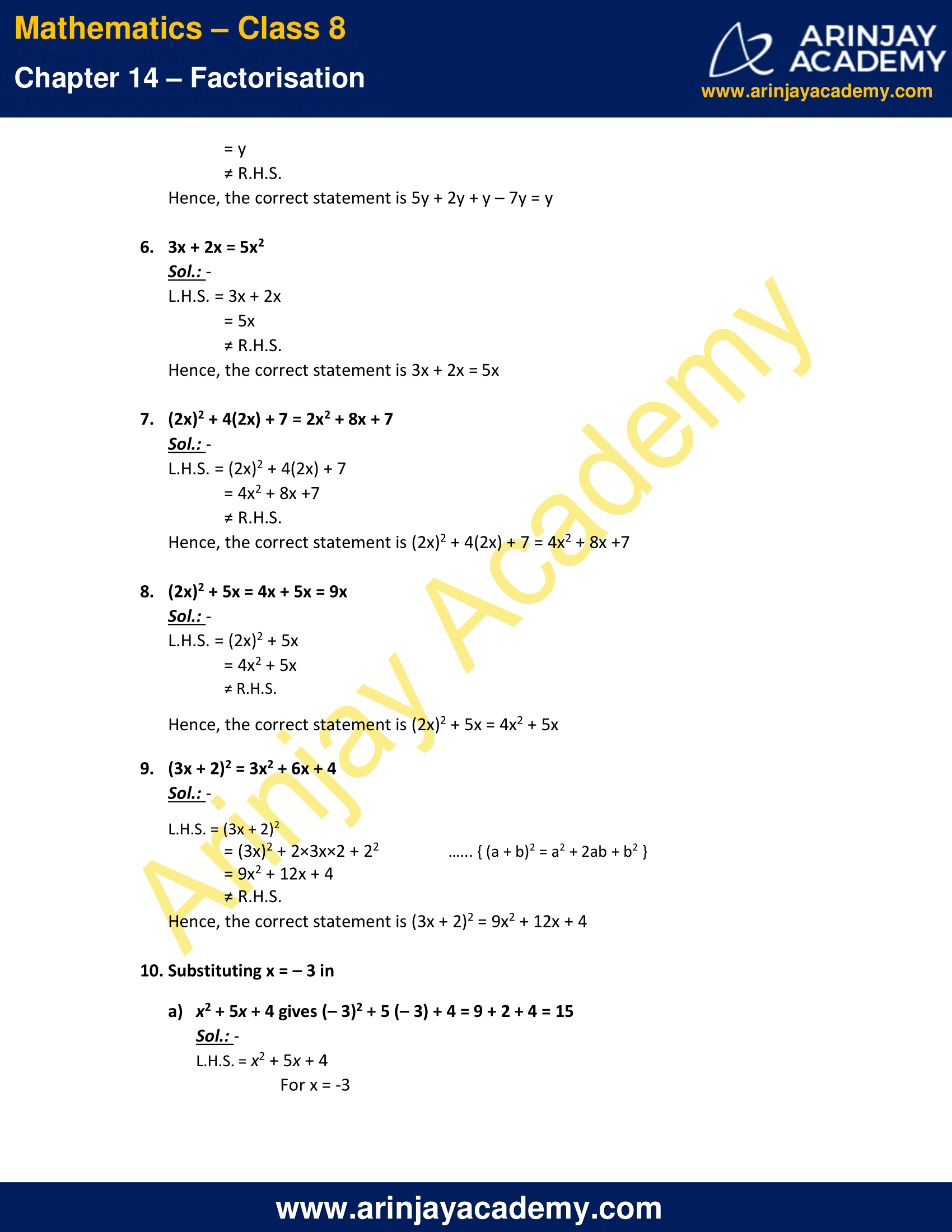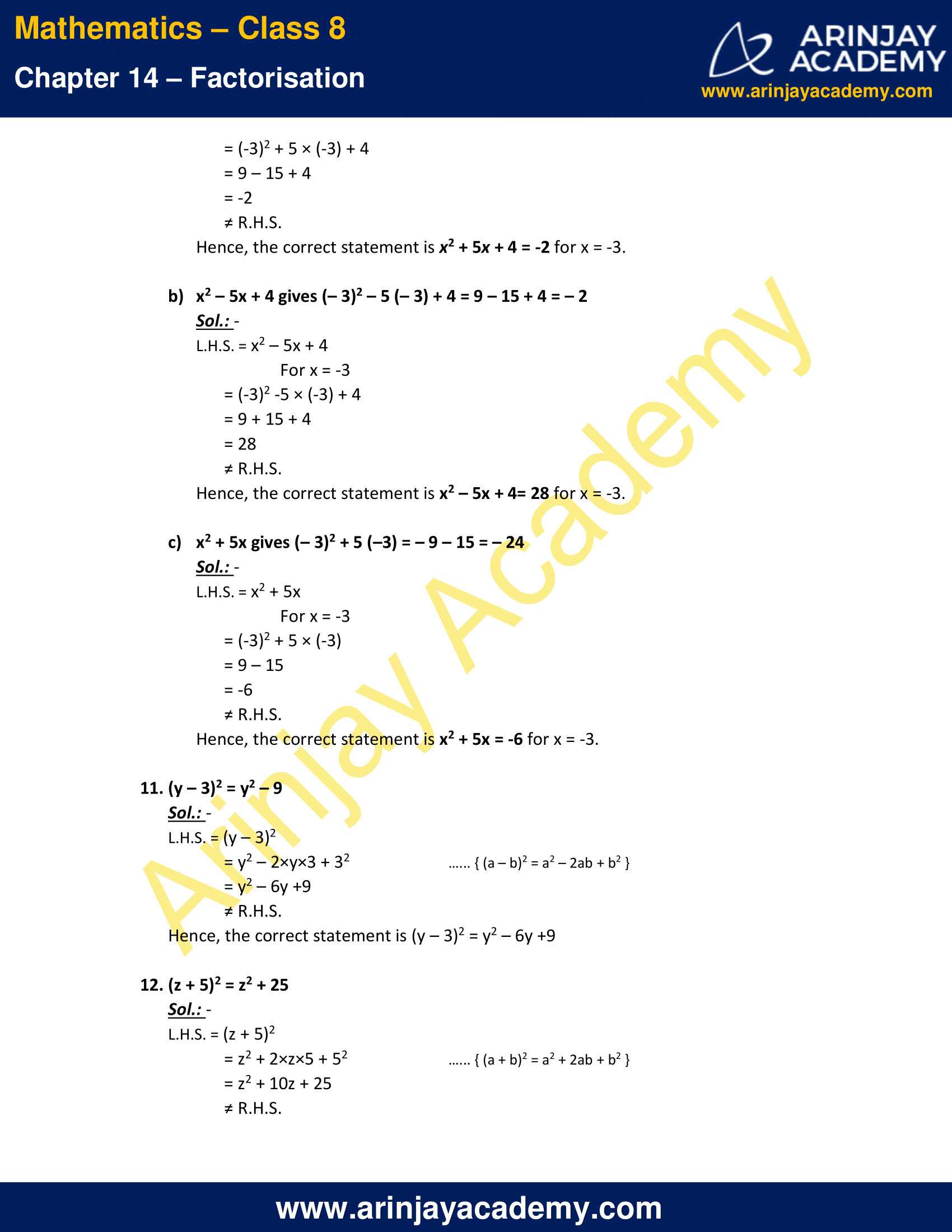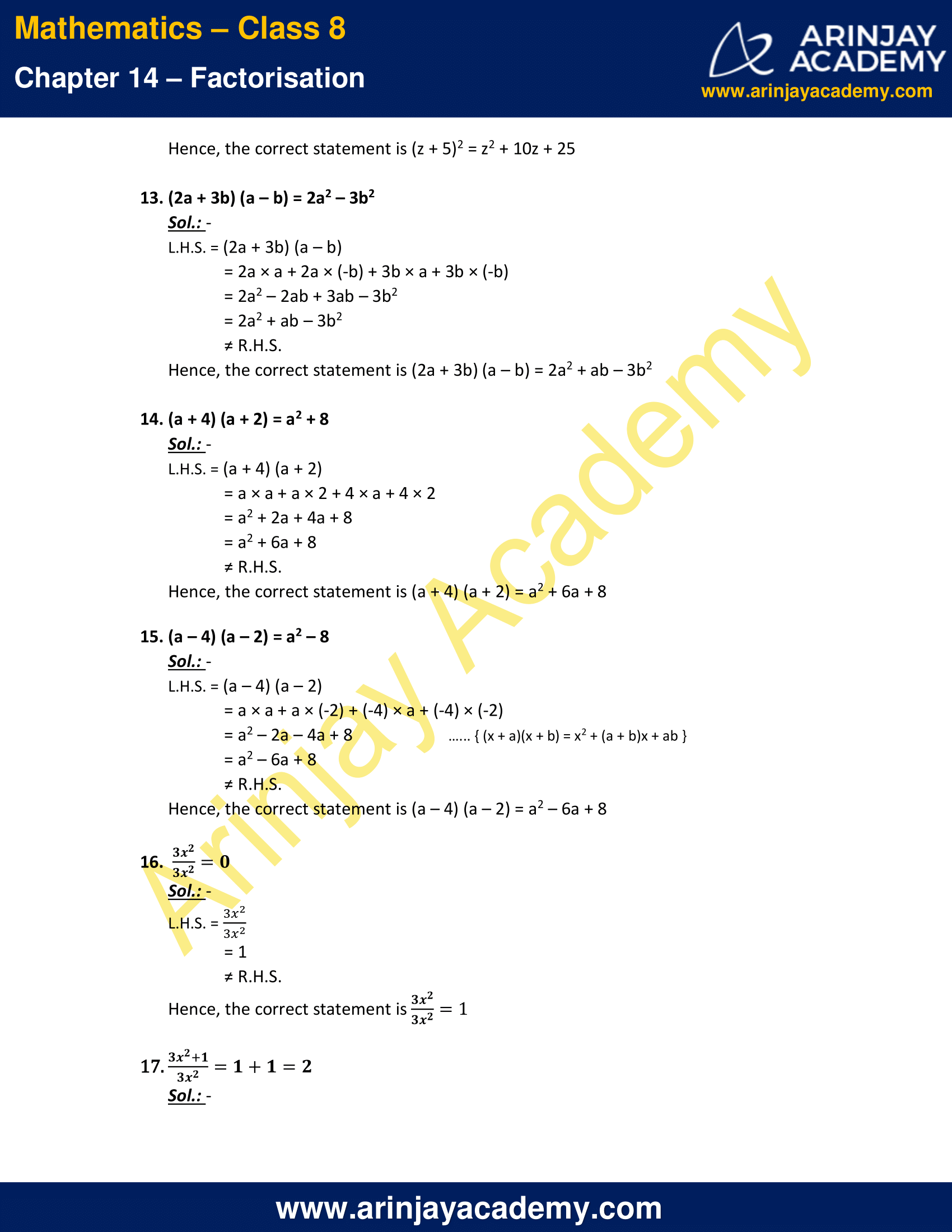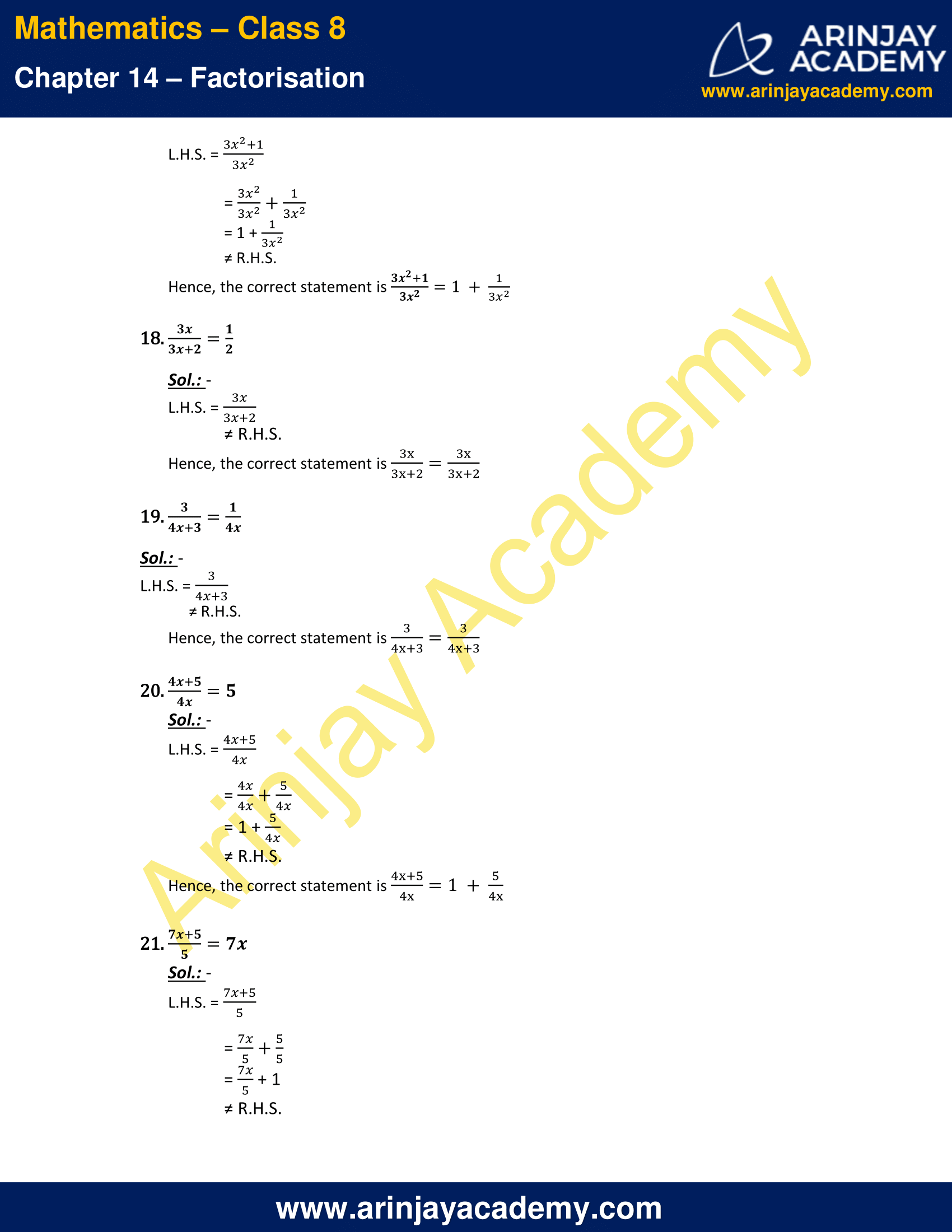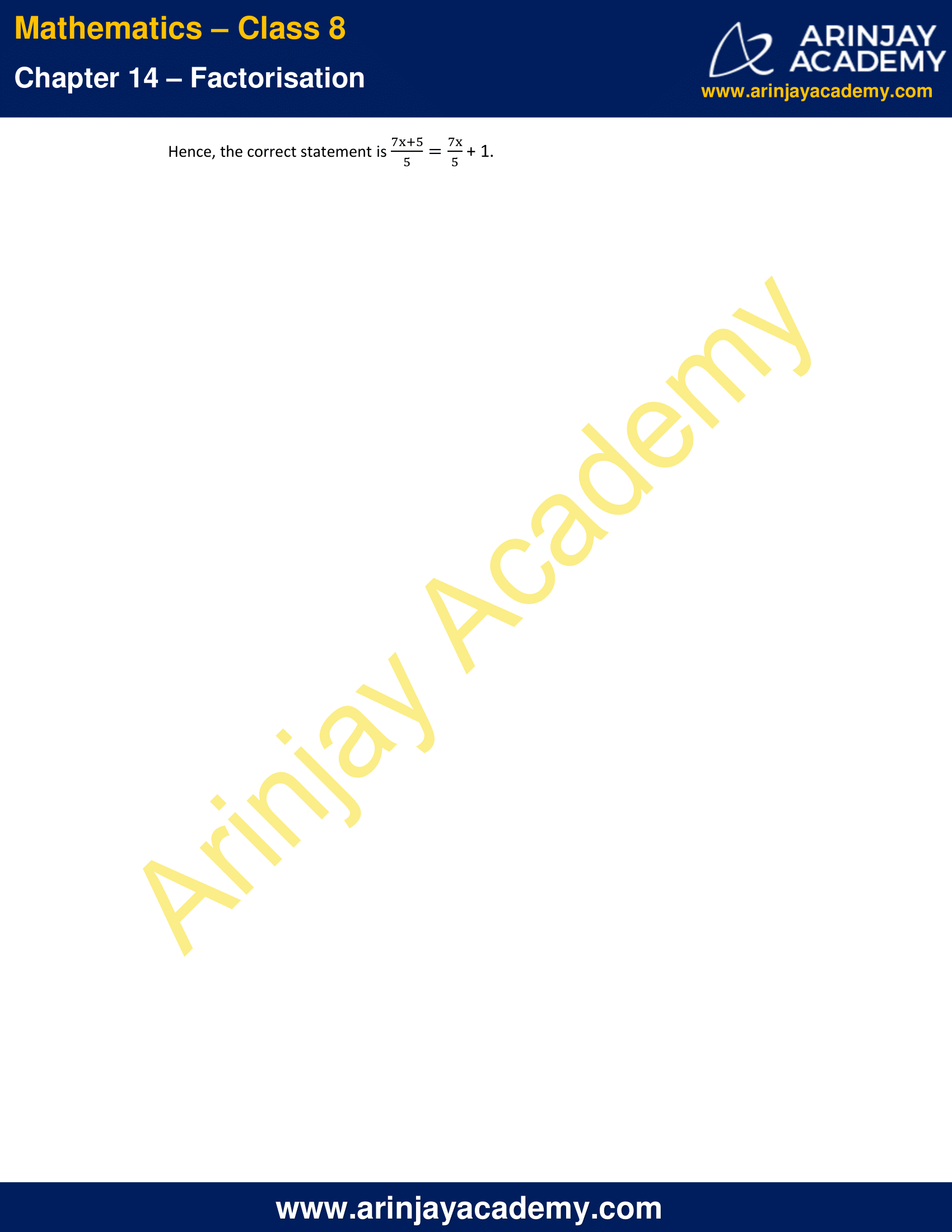NCERT Solutions for Class 8 Maths Chapter 14 Exercise 14.4 – Factorisation

Find and correct the errors in the following mathematical statements.

1. 4(x – 5) = 4x – 5

Sol.:

L.H.S. = 4(x – 5)
= 4×x – 4×5
= 4x – 20
≠ R.H.S.
Hence, the correct statement is 4(x – 5) = 4x – 20

2. x(3x + 2) = 3x2 + 2

Sol.:

L.H.S. = x(3x + 2)
= x×3x + x×2
= 3x2 +2x
≠ R.H.S.
Hence, the correct statement is x(3x + 2) = 3x2 +2x

3. 2x + 3y = 5xy

Sol.:

L.H.S. = 2x + 3y
= 2x + 3y
≠ R.H.S.
Hence, the correct statement is 2x + 3y = 2x + 3y

4. x + 2x + 3x = 5x

Sol.:

L.H.S. = x + 2x + 3x
= 1x + 2x + 3x
= 6x
≠ R.H.S.
Hence, the correct statement is x + 2x + 3x = 6x

5. 5y + 2y + y – 7y = 0

Sol.:

L.H.S. = 5y + 2y + y – 7y
= 5y + 2y + 1y – 7y
= y
≠ R.H.S.
Hence, the correct statement is 5y + 2y + y – 7y = y

6. 3x + 2x = 5x2

Sol.:

L.H.S. = 3x + 2x
= 5x
≠ R.H.S.
Hence, the correct statement is 3x + 2x = 5x

7. (2x)2 + 4(2x) + 7 = 2x2 + 8x + 7

Sol.:

L.H.S. = (2x)2 + 4(2x) + 7
= 4x2 + 8x +7
≠ R.H.S.
Hence, the correct statement is (2x)2 + 4(2x) + 7 = 4x2 + 8x +7

8. (2x)2 + 5x = 4x + 5x = 9x

Sol.:

L.H.S. = (2x)2 + 5x
= 4x2 + 5x
≠ R.H.S.
Hence, the correct statement is (2x)2 + 5x = 4x2 + 5x

9. (3x + 2)2 = 3x2 + 6x + 4

Sol.:

L.H.S. = (3x + 2)2
= (3x)2 + 2×3x×2 + 22   .. { (a + b)2 = a2 + 2ab + b2 }
= 9x2 + 12x + 4
≠ R.H.S.
Hence, the correct statement is (3x + 2)2 = 9x2 + 12x + 4

10. Substituting x = – 3 in

a) x2 + 5x + 4 gives (– 3)2 + 5 (– 3) + 4 = 9 + 2 + 4 = 15

Sol.:

L.H.S. = x2 + 5x + 4
For x = -3
= (-3)2 + 5 × (-3) + 4
= 9 – 15 + 4
= -2
≠ R.H.S.
Hence, the correct statement is x2 + 5x + 4 = -2 for x = -3.

b) x2 – 5x + 4 gives (– 3)2 – 5 (– 3) + 4 = 9 – 15 + 4 = – 2

Sol.:

L.H.S. = x2 – 5x + 4
For x = -3
= (-3)2 -5 × (-3) + 4
= 9 + 15 + 4
= 28
≠ R.H.S.
Hence, the correct statement is x2 – 5x + 4= 28 for x = -3.

c) x2 + 5x gives (– 3)2 + 5 (–3) = – 9 – 15 = – 24

Sol.:

L.H.S. = x2 + 5x
For x = -3
= (-3)2 + 5 × (-3)
= 9 – 15
= -6
≠ R.H.S.
Hence, the correct statement is x2 + 5x = -6 for x = -3.

11. (y – 3)2 = y2 – 9

Sol.:

L.H.S. = (y – 3)2
= y2 – 2×y×3 + 32  .. { (a – b)2 = a2 – 2ab + b2 }
= y2 – 6y +9
≠ R.H.S.
Hence, the correct statement is (y – 3)2 = y2 – 6y +9

12. (z + 5)2 = z2 + 25

Sol.:

L.H.S. = (z + 5)2
= z2 + 2×z×5 + 52  .. { (a + b)2 = a2 + 2ab + b2 }
= z2 + 10z + 25
≠ R.H.S.
Hence, the correct statement is (z + 5)2 = z2 + 10z + 25

13. (2a + 3b) (a – b) = 2a2 – 3b2

Sol.:

L.H.S. = (2a + 3b) (a – b)
= 2a × a + 2a × (-b) + 3b × a + 3b × (-b)
= 2a2 – 2ab + 3ab – 3b2
= 2a2 + ab – 3b2
≠ R.H.S.
Hence, the correct statement is (2a + 3b) (a – b) = 2a2 + ab – 3b2

14. (a + 4) (a + 2) = a2 + 8

Sol.:

L.H.S. = (a + 4) (a + 2)
= a × a + a × 2 + 4 × a + 4 × 2
= a2 + 2a + 4a + 8
= a2 + 6a + 8
≠ R.H.S.
Hence, the correct statement is (a + 4) (a + 2) = a2 + 6a + 8

15. (a – 4) (a – 2) = a2 – 8

Sol.:

L.H.S. = (a – 4) (a – 2)
= a × a + a × (-2) + (-4) × a + (-4) × (-2)
= a2 – 2a – 4a + 8   .. { (x + a)(x + b) = x2 + (a + b)x + ab }
= a2 – 6a + 8
≠ R.H.S.
Hence, the correct statement is (a – 4) (a – 2) = a2 – 6a + 8

16.$\cfrac { { 3x }^{ 2 } }{ { 3x }^{ 2 } }$ = 0

Sol.:

L.H.S. =$\cfrac { { 3x }^{ 2 } }{ { 3x }^{ 2 } }$
= 1
≠ R.H.S.
Hence, the correct statement is$\cfrac { { 3x }^{ 2 } }{ { 3x }^{ 2 } }$ = 1

17.$\cfrac { { 3x }^{ 2 }+1 }{ { 3x }^{ 2 } }$ = 1+1 = 2

Sol.:

L.H.S. =$\cfrac { { 3x }^{ 2 }+1 }{ { 3x }^{ 2 } }$
=$\cfrac { { 3x }^{ 2 } }{ { 3x }^{ 2 } }$ +$\cfrac { 1 }{ { 3x }^{ 2 } }$
= 1 +$\cfrac { 1 }{ { 3x }^{ 2 } }$
≠ R.H.S.
Hence, the correct statement is$\cfrac { { 3x }^{ 2 }+1 }{ { 3x }^{ 2 } }$ = 1 +$\cfrac { 1 }{ { 3x }^{ 2 } }$

18.$\cfrac { { 3x } }{ { 3x+2 } }$ =$\cfrac { 1 }{ 2 }$

Sol.:

L.H.S. =$\cfrac { { 3x } }{ { 3x+2 } }$
≠ R.H.S.
Hence, the correct statement is$\cfrac { { 3x } }{ { 3x+2 } }$ =$\cfrac { { 3x } }{ { 3x+2 } }$

19.$\cfrac { { 3 } }{ { 4x+3 } }$ =$\cfrac { 1 }{ 4x }$

Sol.:

L.H.S. =$\cfrac { { 3 } }{ { 4x+3 } }$
≠ R.H.S.
Hence, the correct statement is$\cfrac { { 3 } }{ { 4x+3 } }$ =$\cfrac { { 3 } }{ { 4x+3 } }$

20.$\cfrac { 4x+5 }{ { 4x } }$ = 5

Sol.:

L.H.S. =$\cfrac { 4x+5 }{ { 4x } }$
=$\cfrac { 4x }{ { 4x } }$ +$\cfrac { 5 }{ { 4x } }$
= 1 +$\cfrac { 5 }{ { 4x } }$
≠ R.H.S.
Hence, the correct statement is

21.$\cfrac { 7x+5 }{ { 5 } }$ = 7x

Sol.:

L.H.S. =$\cfrac { 7x+5 }{ { 5 } }$
=$\cfrac { 7x }{ { 5 } }$ +$\cfrac { 5 }{ { 5 } }$
=$\cfrac { 7x }{ { 5 } }$ + 1
≠ R.H.S.
Hence, the correct statement is$\cfrac { 7x+5 }{ { 5 } }$ =$\cfrac { 7x }{ { 5 } }$ + 1.

The next Exercise for NCERT Solutions for Class 8 Maths Chapter 15 Exercise 15.1 – Introduction to Graphs can be accessed by clicking here

Download NCERT Solutions for Class 8 Maths Chapter 14 Exercise 14.4 – Factorisation